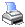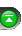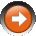﻿ Formatting numbers
 NinjaScript > Educational Resources > Tips > Formatting numbersString formatting on numbers is very useful for creating readable output. This can be done through the use of the number object’s ToString() method.

A common practice is printing out mathematical operations with the use of the ToString() method on the double object. What usually happens is the printing of a long string containing all the decimal places existing in the double. This sometimes makes output cluttered and hard to read. Luckily, C# has a robust set of string formatting options available to make the string more comprehendible.

Here is a list of common formatting options available in the ToString() method:

 double c = 10.25693; Print("No formatting: " + c.ToString()); Print("Currency formatting: " + c.ToString("C")); Print("Exponential formatting: " + c.ToString("E")); Print("Fixed-point formatting: " + c.ToString("F2")); Print("General formatting: " + c.ToString("G")); Print("Percent formatting: " + c.ToString("P0")); Print("Formatted to 2 decimal places: " + c.ToString("N2")); Print("Formatted to 3 decimal places: " + c.ToString("N3")); Print("Formatted to 4 decimal places: " + c.ToString("N4"));

The corresponding output is as follows:

 double c = 10.25693; Print("No formatting: " + c.ToString()); Print("Currency formatting: " + c.ToString("C")); Print("Exponential formatting: " + c.ToString("E")); Print("Fixed-point formatting: " + c.ToString("F2")); Print("General formatting: " + c.ToString("G")); Print("Percent formatting: " + c.ToString("P0")); Print("Formatted to 2 decimal places: " + c.ToString("N2")); Print("Formatted to 3 decimal places: " + c.ToString("N3")); Print("Formatted to 4 decimal places: " + c.ToString("N4"));

For custom formatting you can use the following:

 double phoneNumber = 9162031022; Print("Phone number: " + phoneNumber.ToString("(###) ### - ####"));

Corresponding output:

 Phone number: (916) 203 - 1022

For more information on general string formatting this guide may be of use. Many other resources can be found online through a Google search as well.